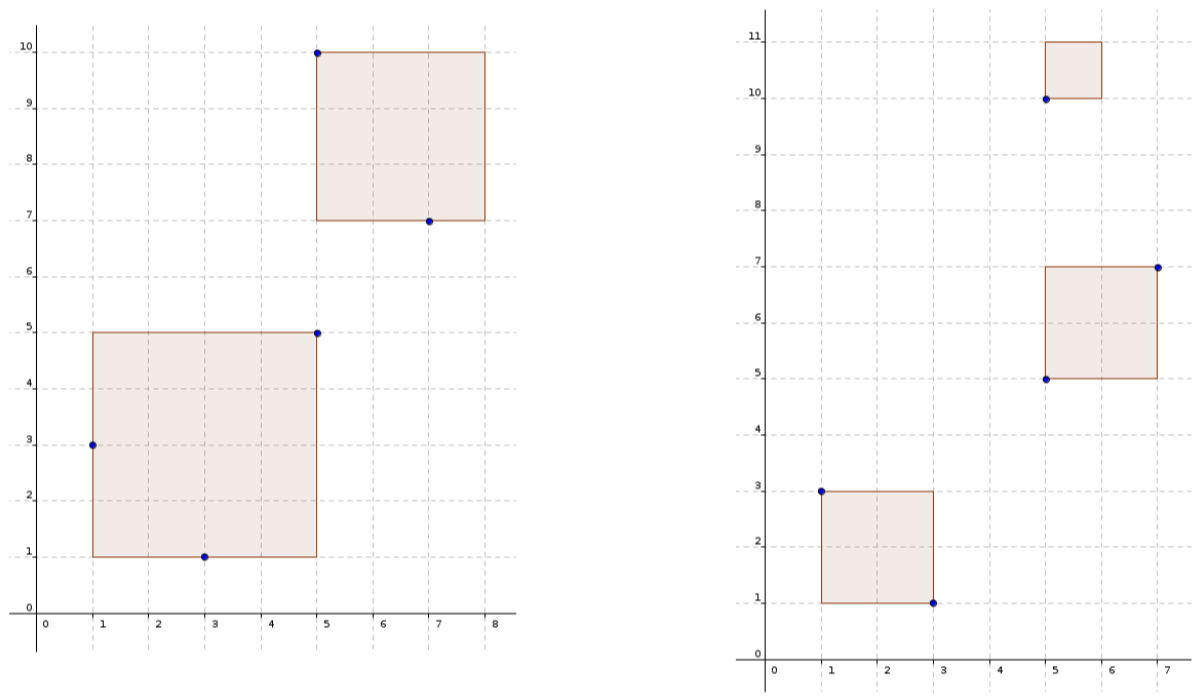시간 제한 메모리 제한 제출 정답 맞은 사람 정답 비율
2.5 초 512 MB 0 0 0 0.000%

## 문제

It is a well-known fact that a group of Russian scientists discovered an alien civilization back in 2016. Their satellite managed to capture $K$ high-resolution square images that forever changed the course of human history. Today, half a decade later, almost every part of the world has its own space program that investigates aliens. Alas, in Croatia we have decided to tackle some more important problems, which leaves scientific research on the shoulders of few enthusiasts. Vladimir is one of them.

Unfortunately, Vladimir doesn’t have enough resources for a spacecraft or an expensive telescope, but he can afford a hot-air balloon and a mobile phone. Instead of an alien civilization, he decided to search for signs of intelligent life on his home planet. Looking down from the balloon, Vladimir notices exactly $N$ people. He decided to capture exactly $K$ square images of average resolution such that each person is seen on exactly one image. Also, he doesn’t want any detail to be visible on more than one image. Furthermore, he finds it important that the largest area visible on some picture is as small as possible.

Vladimir is not a great programmer, so he sent you a formal specification and awaits your help.

Formal specification

There are $N$ integer points in the coordinate system. You need to find exactly $K$ disjunct squares that cover all $N$ points. The squares must have sides parallel with the coordinate axes and their vertices should lie on integer coordinates. The area of the largest square needs to be as small as possible.

We say that a square covers a point if the point is fully inside it or lies on its vertices or sides. Two squares are disjunct if their sides doesn’t intersect or touch, and neither of the squares is fully contained in the other square.

## 입력

The first line contains integers $N$ and $K$ from the task description.

The $i$-th of the next $N$ lines contains integers $x_i$ and $y_i$ ($0 \le |x_i|, |y_i| \le 10^9$) that represent the coordinates of the $i$-th point (person). All $N$ points will be different.

## 출력

The $i$-th of the $K$ lines should contain three integers $x_i$, $y_i$ ($0 \le |x_i|, |y_i| \le 3 \cdot 10^9$) and $l_i$ ($1 \le l_i \le 2 \cdot 10^9$), that uniquely define the location of the $i$-th square, such that the point ($x_i$, $y_i$) denotes its lower-left vertex, and $l_i$ denotes its side length.

If there are multiple correct solutions, output any of them.

## 서브태스크

번호 배점 제한
1 5

$1 \le N \le 100~000, K = 1$

2 21

$1 \le N \le 100~000, K = 2$

3 12

$1 \le N \le 12, K = 3$

4 30

$1 \le N \le 1~000, K = 3$

5 32

$1 \le N \le 100~000, K = 3$

## 예제 입력 1

3 1
1 1
1 3
2 2


## 예제 출력 1

0 1 2


## 예제 입력 2

5 2
1 3
3 1
5 5
5 10
7 7


## 예제 출력 2

1 1 4
5 7 3


## 예제 입력 3

5 3
1 3
3 1
5 5
5 10
7 7


## 예제 출력 3

1 1 2
5 5 2
5 10 1


## 힌트

Explanation of the second and third example:## 채점 및 기타 정보

• 예제는 채점하지 않는다.